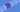# JavaScript - Comparisons

## Comparisons

You may remember from your Math classes that you can use comparison operations to compare variables in an equation. Programming languages let you do this as well so you can add logic to your programs.

``````console.log(2 > 3); // false
console.log(2 < 3); // true
console.log(2 == 4); // false
console.log(2 != 4); // false

console.log('a' != 'b'); // true

const myAge = 24;

console.log(myAge > 23); // true``````

As you can see, we are able to compare variables to one another. The comparison then gives us a boolean value of either true or false.

## String Equality

In JavaScript (and some other languages) there is a difference between `==` and `===` . The latter is called a strict equality. Let's look at some examples of how they differ.

`````` console.log(0 == false); // true
console.log('' == false); // true

console.log(0 === false); // false
console.log('' === false); // false``````

Here you can see that when we use `===` we get false for the comparison. In general, it is best to use the strict equality operator `===` . There are times when you want to use the less strict equality (we will explore later), but always start with the strict (just like const) first.

## Using comparisons to control

Okay, let's finish this lesson out with an example of how to use these in practice. If you don't know the `if` statement, don't worry. It should read pretty straightforward.

``````const age = 17;

if (age < 18) {
console.log('You are to young to vote')
}``````

And there you go! There is much more you can do with comparisons, but this should get you started on writing a lot of custom logic in your programs.

GoTea - KoalaTea